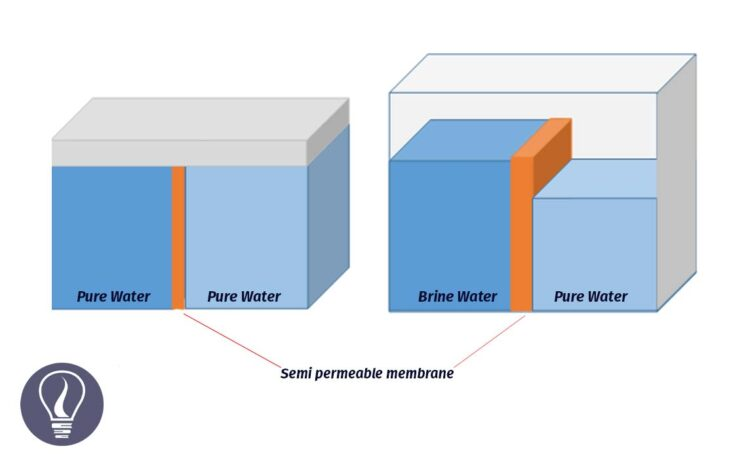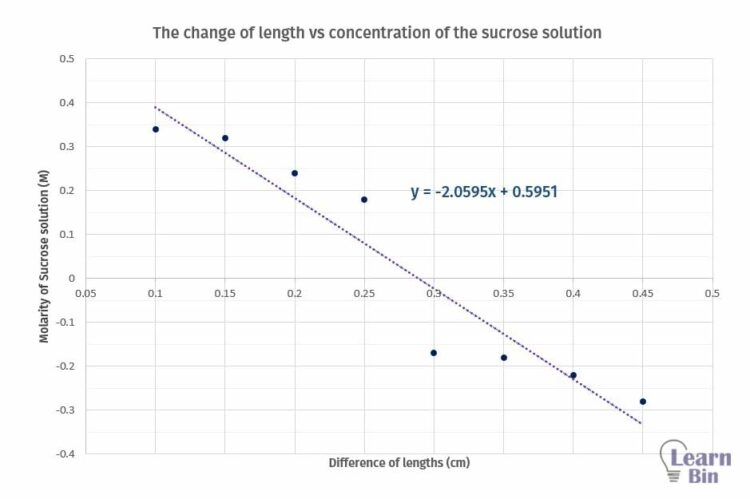More results...

Generic selectors
Exact matches only
Search in title
Search in content
Post Type Selectors

# Water Potential - Water Transport in PlantsB.Sc (Hons, USJ) (Polymer Science and Technology, Chemistry, Physics)
Categories
Last Updated On : September 5, 2023
Published Date : August 1, 2023

Water potential is a measure of the kinetic energy of water molecules. The kinetic energy capacity of water molecules to move from one place to another place is called water potential.

The units of water potential (ψw) are similar to the units of pressure. This can be measured using

• atm (standard atmosphere)
• Pa (Pascal - SI unit)
• Nm-2 (Newtons per square meter)

The water potential of pure water at standard temperature and pressure is zero. This is the highest ψw. When the ψw is decreasing, the minus value of the ψw is increasing. There are several factors that affect the ψw. Factors that affect the ψw are soluble constituents, external pressure, and hydrophilic components.

## Solute potential (ψs)

The change in ψw due to solute components is called solute potential. Due to the solute components, ψw is decreasing. Therefore, the solute potential is always negative.

## Pressure potential (ψp)

The change in ψw due to external pressure is called pressure potential. Due to the pressure, ψw is increasing. Therefore, the pressure potential is always positive.

## Matric potential (ψm)

The change in ψw due to the hydrophilic components is called Matric potential. Matric potential is always negative. That means, due to the hydrophilic substances the water potential will decrease.

By considering solute potential, pressure potential, and matric potential we can write an equation for water potential as follows.

## Water potential of a plant cell

In a turgid cell, the vacuole and the cytoplasm get bigger due to the higher water content. This creates pressure against the cell wall. This pressure which is created by the protoplast is known as the Turgid pressure. (Protoplast is the living part of the cell without a cell wall).

The cell wall creates an equal and opposite pressure to the turgid pressure on the protoplast. This pressure is called "Wall pressure". Due to the wall pressure, there is a pressure potential in the cell. Also, there is a solute potential due to the solute content in the vacuole.

Therefore, the water potential equation for a plant cell can be written as follows.

## Water potential example questions and solutions - How to solve questions related to water potential

Question 01

There are two cells A and B in contact with each other. The pressure potentials of A and B are +500 kPa and +400 kPa respectively. The solute potential of A is -1800 kPa. The water potential of B is -1600 kPa.

1. Find the water potential of cell A.
2. Find the solute potential of cell B
3. What is the net osmosis direction?
4. Find the water potential of two cells at the equilibrium.
5. By assuming that the solute potential does not change in cell B, find the pressure potential at the equilibrium.

Solution

Solution for part 01

Solution for part 02

Solution for part 03

Cell A has a higher water potential. Therefore, water molecules travel from cell A to cell B. Net osmosis direction is A to B.

Solution for part 04

Solution for part 05

## Determination of the water potential of potato tuber

The water potential of a potato tuber can be determined by the tissue volume method. When plant tissue is placed in a solution, osmosis is occurred according to the water potentials of the solution and the plant tissue. If the water potential is higher in the plant tissue than in the solution, water molecules are removed from the plant tissue.

Therefore, the volume of the tissue is decreased. If the water potential is lower in the issue than the solution, water molecules enter the tissue, and the volume of the tissue is increased. When the water potentials are the same in both solution and plant tissue, the net osmosis is zero.

In this situation, we assume that the solute potentials of both solution and the plant tissue are the same. According to this theory, we can find the water potential of the plant tissue.

### Reagents

A series of sucrose solutions with different molarities. (0.1 M, 0.15 M, 0.2 M, 0.25 M, 0.3 M, 0.35 M, 0.4 M, 0.45 M)

### Method

• Cylindrical sections of potato tuber are prepared using a cork borer. Cut the potato sections into sections 2 - 5 cm in length.
• Immerse the potato sections in the prepared sucrose solution.  Immerse two potato tubers per solution.
• Incubate the samples for 1 - 1.5 hours to become equilibrium.
• After 1 - 1.5 hours measure the length of each potato section using a Vernier caliper. And take the difference between the initial length and the final length.

Plot a graph of the change of length with respect to the concentration of the sucrose solution.Graph 01: Determination of the water potential of potato tuber - Graph of the change of length vs concentration of the sucrose solution

Molarity of the sucrose solution, that there is no change in length. (at this point y = 0)

From the graph, we can determine the concentration where there is no length change. If there is no change in length means there is no net osmosis occurring at that concentration. Using standard tables, we can find the solute potential of the tissue.## References and Attributes

### Figures:

The cover image was designed using an image by Wikilakz, licensed under CC BY-SA 4.0, via Wikimedia Commons